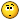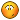# [SOLVED] MasterEffect OpenGL matrix swizzeling problem

• Mikan
•Topic Author
• Offline
• Posts: 14
5 years 4 months ago - 5 years 4 months ago #1 by Mikan
Hi again^^
Amnesia the Dark Decent would not accept this part of SSAOgen, not sure why.. code looks good and work on other games```float3x3 matRotate;

float hao = 1.0f / (1.0f + vRotation.z);

matRotate._m00 =  hao * vRotation.y * vRotation.y + vRotation.z;
matRotate._m01 = -hao * vRotation.y * vRotation.x;
matRotate._m02 = -vRotation.x;
matRotate._m10 = -hao * vRotation.y * vRotation.x;
matRotate._m11 =  hao * vRotation.x * vRotation.x + vRotation.z;
matRotate._m12 = -vRotation.y;
matRotate._m20 =  vRotation.x;
matRotate._m21 =  vRotation.y;
matRotate._m22 =  vRotation.z;```

Changed it to this one and it worked fine and should do same I think. Tested with both Amnesia and Borderlands 2 ^^
```float hao = 1.0f / (1.0f + vRotation.z);

float3x3 matRotate = float3x3(float3(  hao * vRotation.y * vRotation.y + vRotation.z,
-hao * vRotation.y * vRotation.x, -vRotation.x ),
float3( -hao * vRotation.y * vRotation.x,
hao * vRotation.x * vRotation.x + vRotation.z, -vRotation.y ),
float3( vRotation.x, vRotation.y, vRotation.z) );```

• crosire
•• Offline
• Posts: 3723
5 years 4 months ago #2 by crosire

Mikan wrote: Hi again^^
Amnesia the Dark Decent would not accept this part of SSAOgen, not sure why.. code looks good and work on other gamesThat's a bug in the current ReShade to GLSL compiler, where I erroneously asumed GLSL supports matrix swizzeling (which actually works on NVIDIA). Fixed in the next version, where it generates working code again. Workaround for now is to use the array operator or the constructor directly (like you did). =)

• Mikan
•Topic Author
• Offline
• Posts: 14
5 years 4 months ago #3 by Mikan

crosire wrote: That's a bug in the current ReShade to GLSL compiler, where I erroneously asumed GLSL supports matrix swizzeling (which actually works on NVIDIA). Fixed in the next version, where it generates working code again. Workaround for now is to use the array operator or the constructor directly (like you did). =)

The amount code you guys are keeping track on most be quite a lotTrying to learn myself.Looking forward to new updates^^